The G3GRID Procedure

# Concepts

The input data set must contain at least three numeric variables:

• two horizontal variables (x, y)

• one or more vertical variables, z through z-n, that is interpolated or smoothed as if it were a function of the two horizontal variables

The G3GRID procedure can process multiple vertical variables for each pair of horizontal variables that you specify:

• if you specify more than one vertical variable, the G3GRID procedure performs a separate analysis, and produces interpolated or smoothed values for each vertical variable

• if more than one observation in the input data set has the same values for both horizontal variables, x and y, only the first observation is used in the interpolation. A warning message is printed to the log.

• by default, the interpolation is performed after both variables are similarly scaled, because the interpolation methods assume that the scales of x and y are comparable

The GRID statement, enables you to name multiple vertical variables (z - z-n), to produce a data set that contains two horizontal variables, and multiple vertical variables. The resulting data set enables you to produce plots of the relationships of the two horizontal variables, to different vertical variables.

If the points that are generated by the horizontal variables tend to lie along a curve, a poor interpolation or spline can result. In such cases, the vertical variable(s), and one of the horizontal variables should be modeled as a function of the remaining horizontal variable. A scatter plot of the two horizontal variables enable you to determine the appropriate function.

If the horizontal variable points are collinear, the procedure interpolates the function as constant, along lines perpendicular to the line in the plane that is generated by the input data points.

The output data set contains:

• the two horizontal variables

• the interpolated or smoothed vertical variables

• any BY variables

G3Grid enables you to control both the number of x and y values in the output data set, and the values themselves. In addition, you can specify an interpolation method.

The G3GRID procedure can use one of three interpolation methods: bivariate interpolation (the default), spline interpolation, and smoothing spline interpolation.

### Bivariate Interpolation

Unless you specify the SPLINE option, the G3GRID procedure is an interpolation procedure. It calculates the z values for x, y points that are missing from the input data set. The surface that is formed by the interpolated data passes precisely through the data points in the input data set.

This method of interpolation works best for fairly smooth functions, with values given at uniformly distributed points in the plane. If the data points in the input data set are erratic, the default interpolated surface can be erratic.

This default method is a modification of that described by Akima (1978). This method consists of the following actions:

1. dividing the plane into non-overlapping triangles that use the positions of the available points

2. fitting a bivariate fifth degree polynomial within each triangle

3. calculating the interpolated values by evaluating the polynomial at each grid point that falls in the triangle

The coefficients for the polynomial are computed based on the following criteria:

• the values of the function at the vertices of the triangle

• the estimated values for the first, and second derivatives of the function at the vertices

The estimates of the first, and second derivatives are computed using the n nearest neighbors of the point, where n is the number specified in the GRID statement's NEAR= option. A Delauney triangulation (Ripley 1981, p. 38), is used for the default method. The coordinates of the triangles are available in an output data set, if requested by the OUTTRI= option, in the PROC G3GRID statement. This is the default interpolation method.

### Spline Interpolation

If you specify the SPLINE option, a method is used that produces either an interpolation. or smoothing that is optimally smooth. See (Harder and Desmarais 1972, Meinguet 1979, Green and Silverman 1994). The surface that is generated can be thought of as one that would be formed if a stiff, thin metal plate were forced through, or near the given data points. For large data sets, this method is substantially more expensive than the default method.

The function u, formed when you specify the SPLINE option, is determined by letting: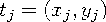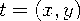and
where
The coefficients c1, c2,..., cn, and d1, d2, d3 of this polynomial are determined by the following equations:
, and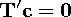where

E

is the n × n matrix E(ti, tj )

I

is the n × n identity matrix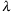is the smoothing parameter that is specified in the SMOOTH= option

c

is (c1 ,..., cn )

z

is (z1 ,..., zn )

d

is (d1, d2, d3)

T

is the n × three matrix whose ith row is (1, xi, yi).

See Wahba (1990) for more detail.

### Spline Smoothing

Using the SMOOTH= option on the GRID statement with the SPLINE option, enables you to produce a smoothing spline. See Eubank (1988) for a general discussion of spline smoothing. The value or values specified in the SMOOTH= option are substituted forin the equation that is described in Spline Interpolation. A smoothing spline trades closeness to the original data points for smoothness. To find a value that produces the best balance between smoothness, and fit to the original data, several values for the SMOOTH= option can be run.Previous Page | Next Page | Top of Page